## MP Board Class 7th Maths Solutions Chapter 2 Fractions and Decimals Ex 2.2

Question 1.
Which of the drawings (a) to (d) show: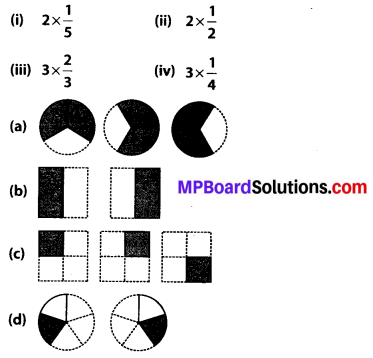Solution:
(i) 2 × $$\frac{1}{5}$$ represents addition of 2 figures, each representing 1 shaded part out of 5 equal parts. Hence, 2 × $$\frac{1}{5}$$ is represented by (d)
(ii) 2 × $$\frac{1}{2}$$ represents addition of 2 figures, each representing 1 shaded part out of 2 equal parts. Hence, 2 × $$\frac{1}{2}$$ is represented by
(b) .
(iii) 3 × $$\frac{2}{3}$$ represents addition of 3 figures, each representing 2 shaded parts out of 3 equal parts. Hence, 3 × $$\frac{2}{3}$$ is represented by (a).
(iv) 3 × $$\frac{1}{4}$$ represents addition of 3 figures, each representing 1 shaded part out of 4 equal parts. Hence, 3 × $$\frac{1}{4}$$ is represented by (c).

Question 2.
Some pictures (a) to (c) are given below. Tell which of them show: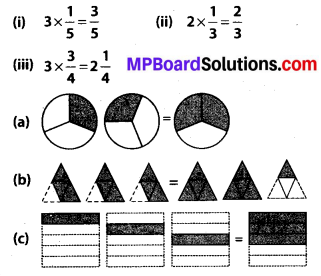Solution:
(i) 3 × $$\frac{1}{5}$$ represents the addition of 3 figures, each representing 1 shaded part out of 5 equal parts and $$\frac{3}{5}$$ represents 3 shaded parts out of 5 equal parts.
Hence, $$3 \times \frac{1}{5}=\frac{3}{5}$$ is represented by (c).

(ii) $$2 \times \frac{1}{3}$$ represents the addition of 3 figures, each representing 1 shaded part out of 3 equal parts and $$\frac{2}{3}$$ represents 2 shaded parts out of 3 equal parts.
Hence, $$2 \times \frac{1}{3}=\frac{2}{3}$$ is represented by (a).

(iii) $$3 \times \frac{3}{4}$$ represents the addition of 3 figures, each representing 3 shaded parts out of 4 equal parts and $$2 \frac{1}{4}$$ represents 2 fully shaded figures and one figure having 1 shaded part out of 4 equal parts.
Hence, $$3 \times \frac{3}{4}=2 \frac{1}{4}$$ is represented by (b).Question 3.
Multiply and reduce to lowest form and convert into a mixed fraction: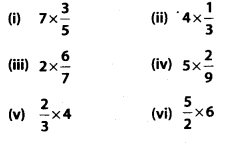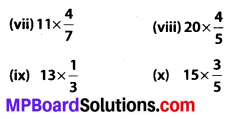Solution: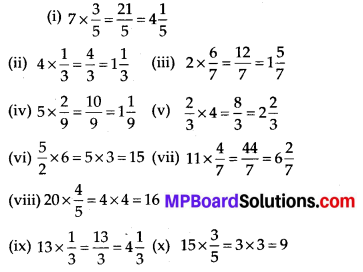Question 4.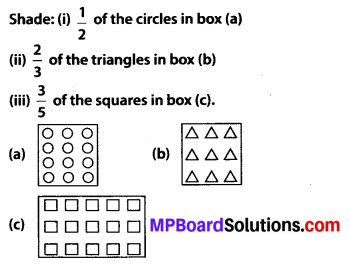Solution:
(i) There are 12 circles in the given box.
(a)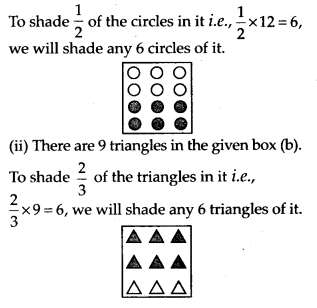(iii) There are 15 squares in the given box (c). To shade $$\frac{3}{5}$$ of the squares in it i.e., $$\frac{3}{5} \times 15=9$$, we will shade any 9 squares of it.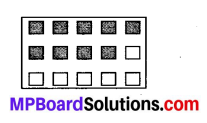Question 5.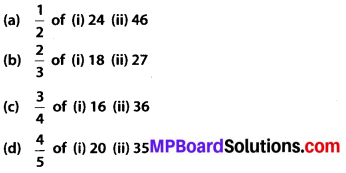Solution: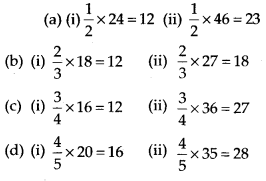Question 6.
Multiply and express as a mixed fraction: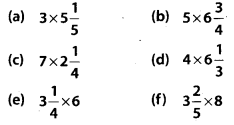Solution: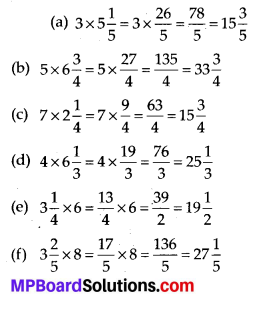Question 7.
Find: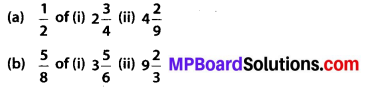Solution: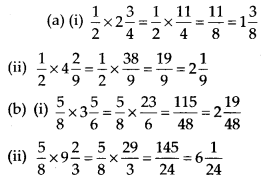Question 8.
Vidya and Pratap went for a picnic. Their mother gave them a water bottle that contained 5 litres of water. Vidya consumed $$\frac{2}{5}$$ of the water. Pratap consumed the remaining water.
(i) How much water did Vidya drink?
(ii) What fraction of the total quantity of water did Pratap drink?
Solution:
(i) Water consumed by Vidya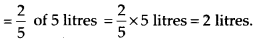(ii) Fraction of remaining water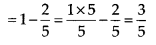Thus, Pratap consumed $$\frac{3}{5}$$ of the total quantity of water.